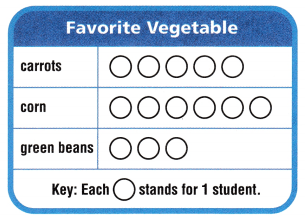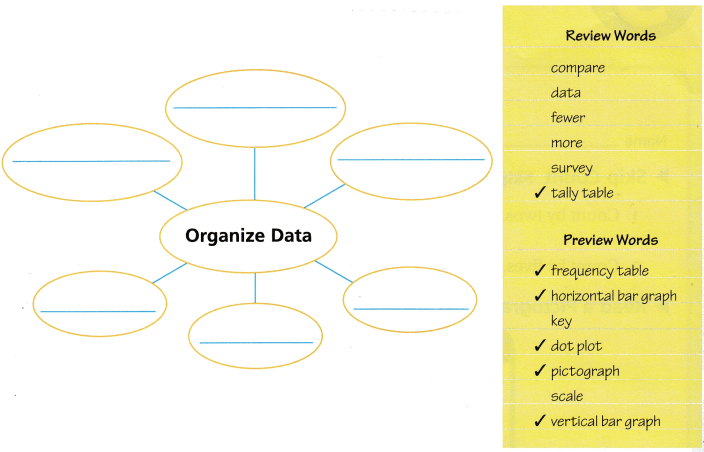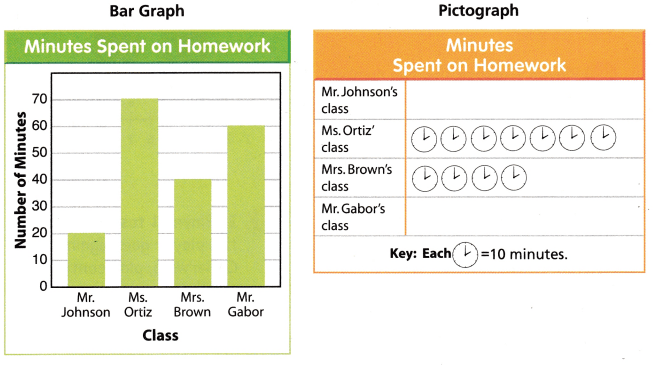# Texas Go Math Grade 3 Unit 5 Answer Key Data Analysis

Refer to our Texas Go Math Grade 3 Answer Key Pdf to score good marks in the exams. Test yourself by practicing the problems from Texas Go Math Grade 3 Unit 5 Answer Key Data Analysis.

## Texas Go Math Grade 3 Unit 5 Answer Key Data Analysis

Show What You Know

Check your understanding of important skills.

Skip Count: Skip count to find the missing numbers.

Question 1.
Count by twos. 2, 4, ______, _______, 10, _______, ________, 16
Explanation:
Multiples of 2 are 2,4,6,8,10,12,16

Count by fives. 5, 10, ______, _______, ____ , 30, ______
Explanation:
Multiples of 5 are 5,10,15,20,25,30,35

Read a Pictograph For 3, use the pictograph.Question 3.
Which vegetable did the most students choose? __________
Explanation:
With the help of bar graph we can say that most of the students opted for Corn

Read a Bar Graph: For 4-5, use the bar graph.Question 4.
How many students chose puzzles? __________ students
Explanation:
With the help of bar graph we can say that 6 students have chosen puzzles

How many students answered the question? __________ students
Explanation:
With the help of bar graph we can say that 16 students have answered the questions

Vocabulary Builder

Visualize It
Complete the bubble map by using the words with a ✓.Explanation:
Bubble map is completed with review words and preview words

Understand Vocabulary

Write the preview word that answers the riddle.

I am a graph that records each piece of data above a number line. ____________
Explanation:
A graph that records each piece of data on a number line.

Question 2.
I am the numbers that are placed at fixed distances on a graph to help label the graph. __________
Explanation:
The numbers placed at fixed distances on a graph to help label the graph.

Question 3.
I am the part of a map or graph that explains the symbols. _____________
The part of a map or graph that explains the symbols.

I am a graph that uses pictures to show and compare the information. ___________
Explanation:
A graph that uses pictures to show and compare information.

Question 5.
I am a table that uses numbers to record data. _____________
Explanation:
A table that uses numbers to record

Vocabulary

To organize and display information, you can use a bar graph or a pictograph.

The teachers at Park Elementary surveyed their students to find out how much time they spent on homework. The bar graph shows their data. Use the bar graph to complete the pictograph.Explanation:
Pictograph was completed.

Writing Survey 5 students about how much time they spent doing homework last night. You can use this information to make a bar graph or a pictograph.Reading Look for this book in the library. Tiger Math: Learning to Graph From a Baby Tiger, by Ann Whitehead Nagda and Cindy Bickel.

Dot Plot Sums
Object of the Game Make a dot plot of the sums you roll.

Materials

• 2 number cubes labeled 1-6
• number lines labeled 2-12
• pencil or marker

Set Up
Each player gets one number line.
Number of Players 2, 3, or 4

How to Play

1. Player 1 tosses the two number cubes. The sum of the numbers tossed is a data value on the dot plot.2. Player 1 draws a dot above the number line for the sum.

3. If Player 1 tosses doubles, the player goes again. Otherwise, play continues clockwise.

4. The first player who has five dots above the same sum wins the game.Answer: This is a number game to learn about the number line

Scroll to Top